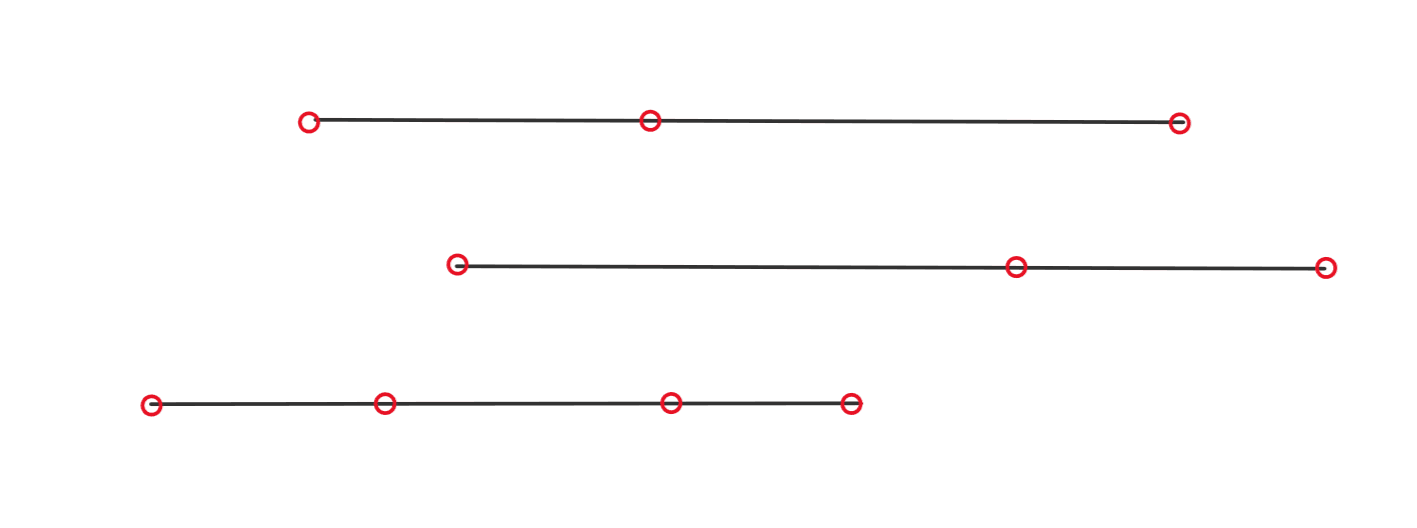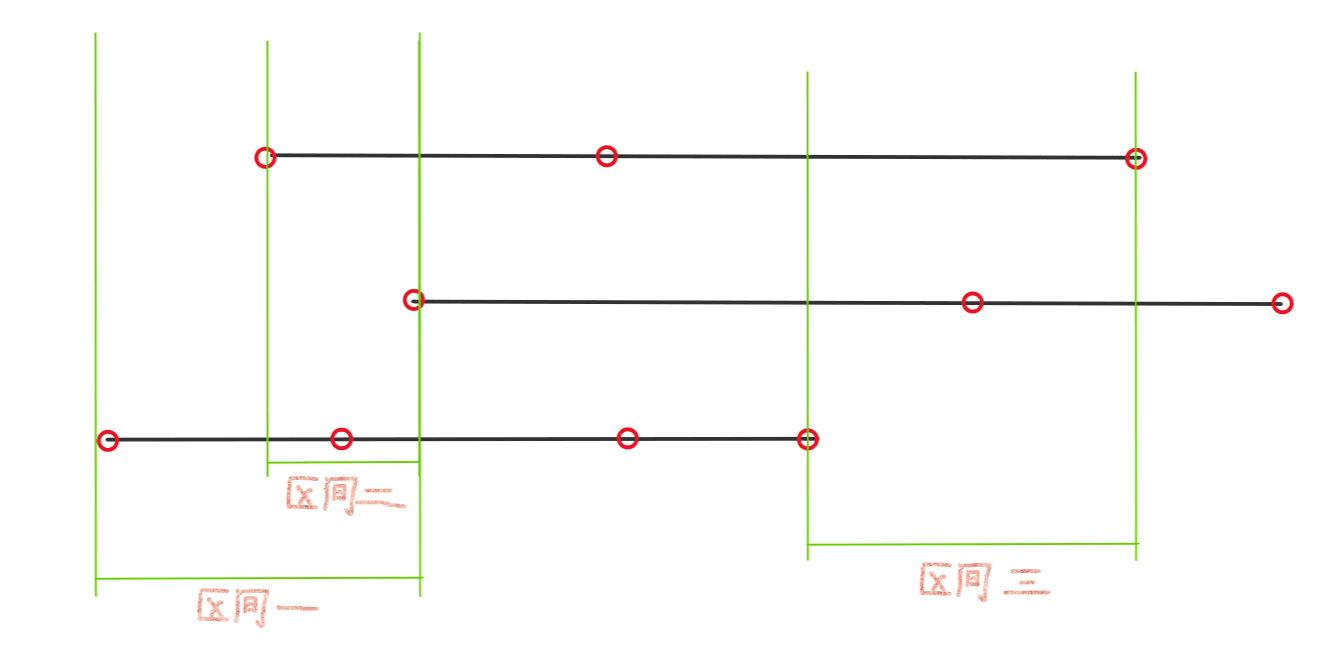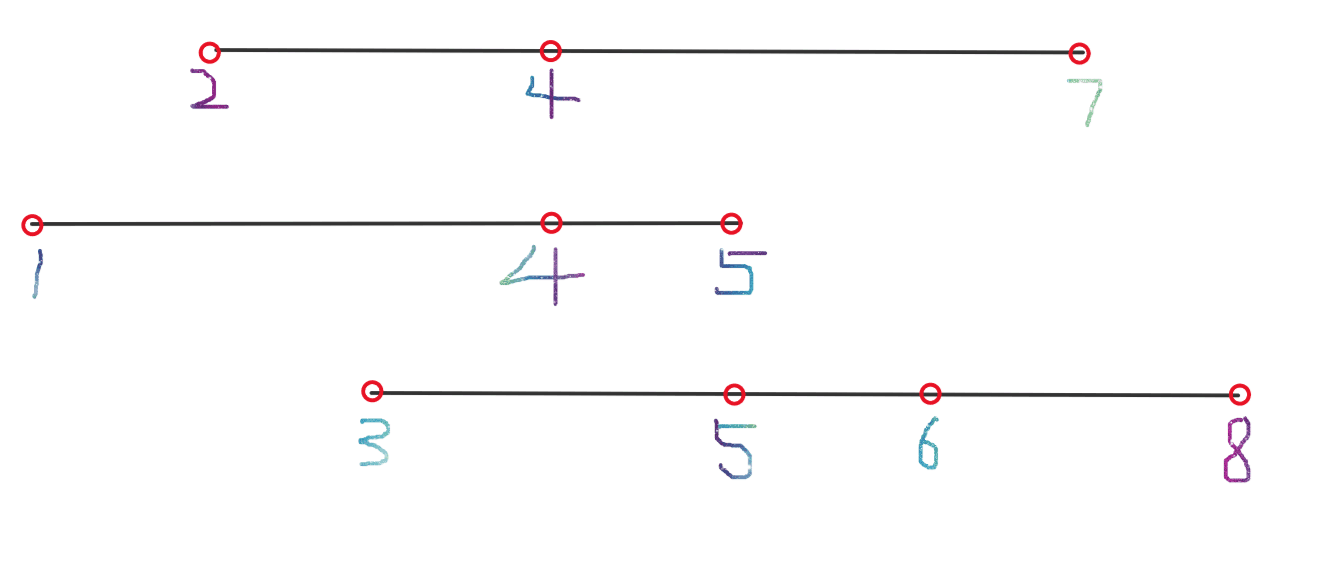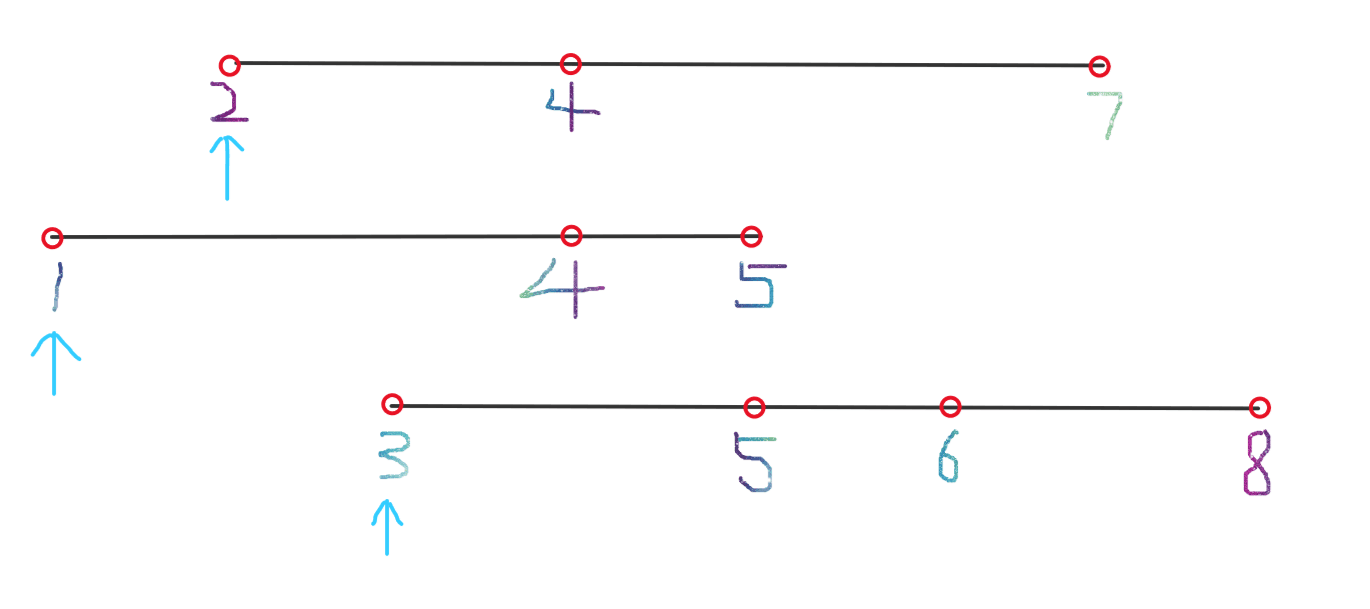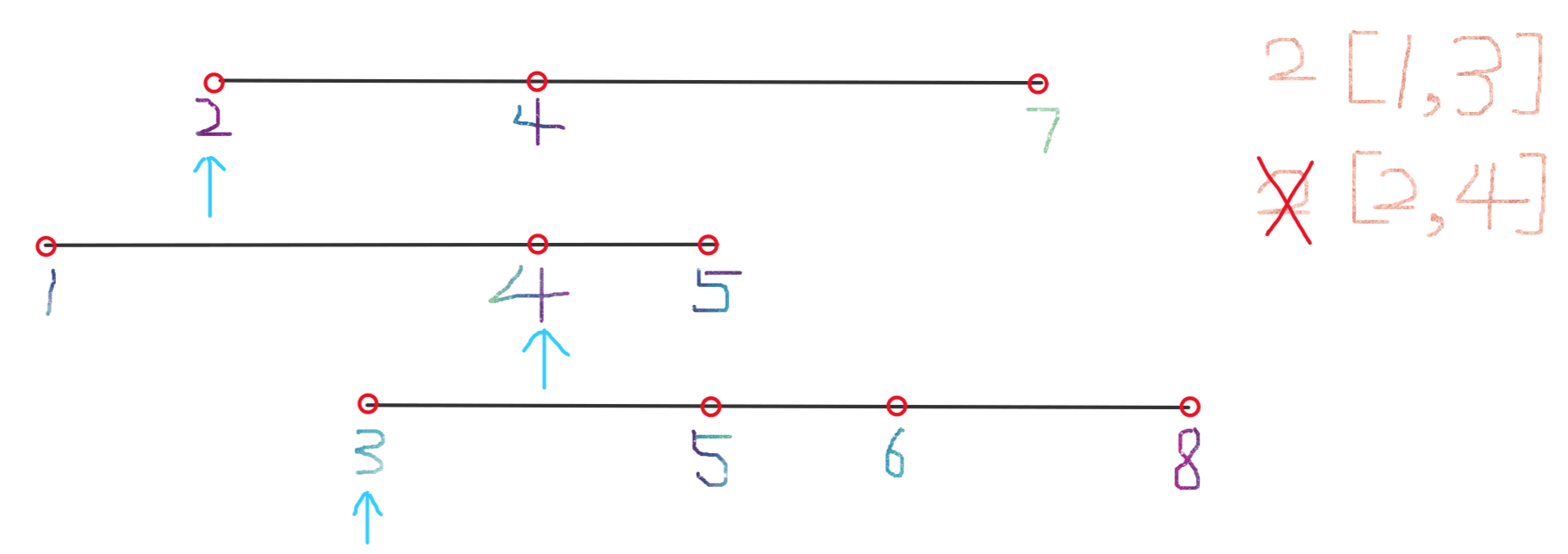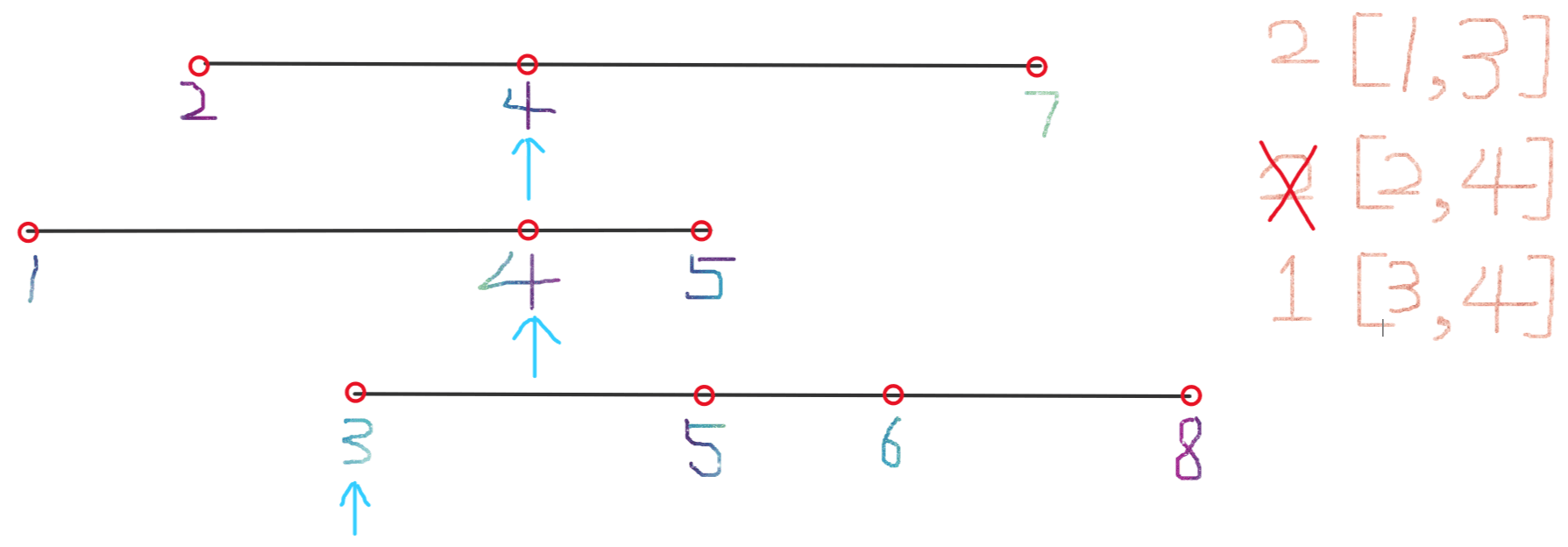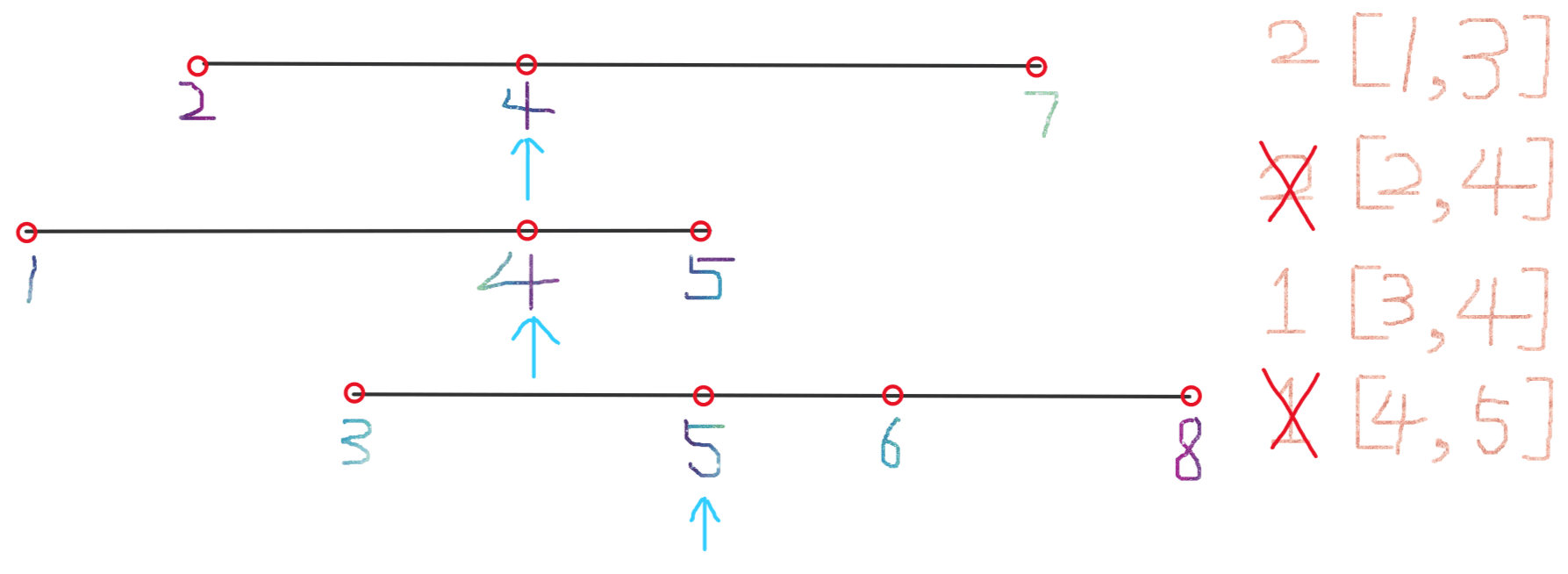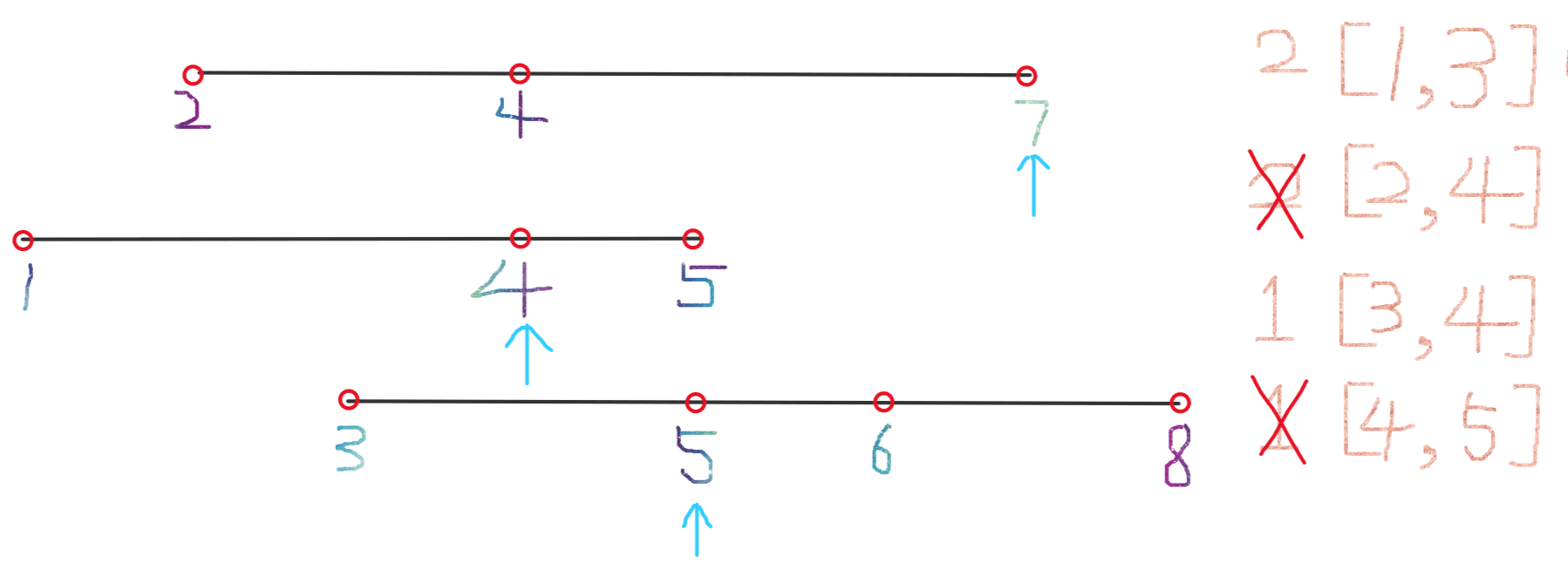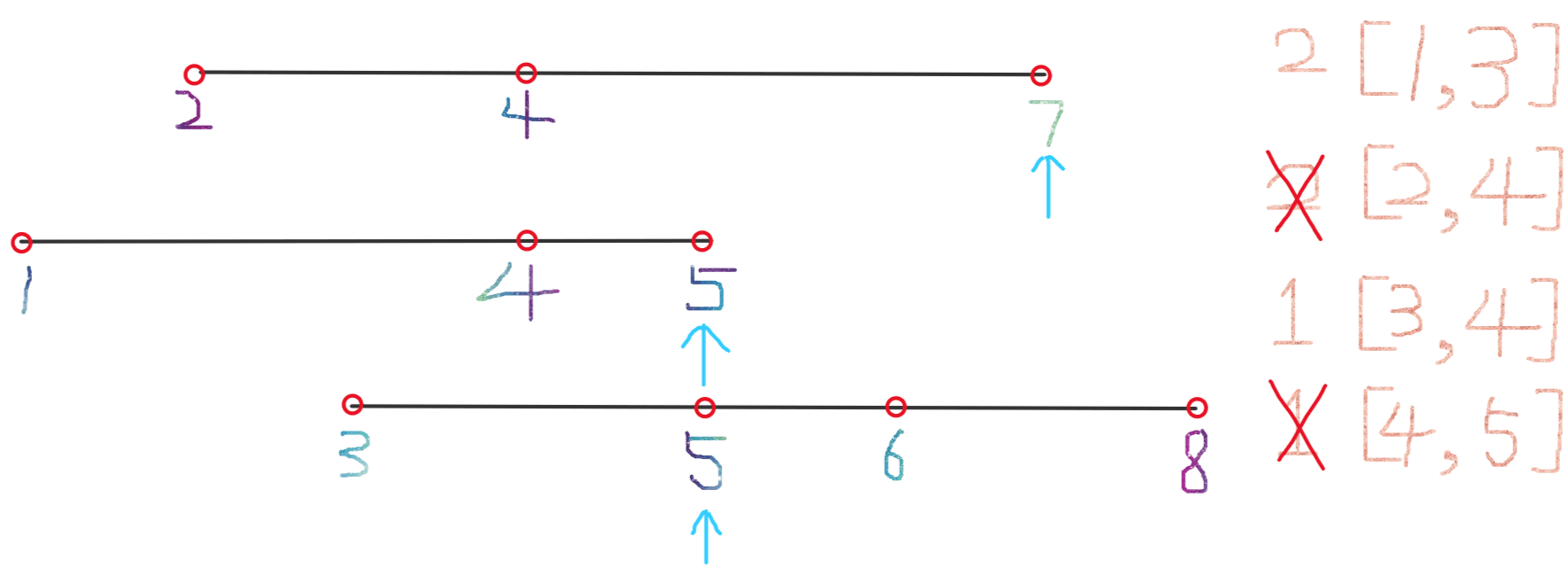# # Leetcode 632. 最小区间

## # 题目描述

• `nums.length == k`
• `1 <= k <= 3500`
• `1 <= nums[i].length <= 50`
• `-105 <= nums[i][j] <= 105`
• `nums[i]` 按非递减顺序排列

## # 解题思路